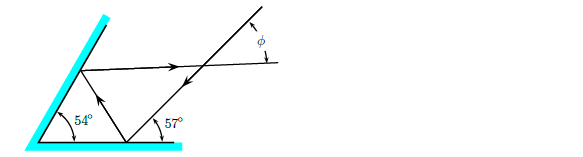# Problem: The reflecting surfaces of two intersecting flat mirrors are at an angle of 54°, as shown in the figure. A light ray strikes the horizontal mirror at an angle of 57° respect to the mirror’s surface. Calculate the angle Φ.

80% (42 ratings)
###### Problem Details

The reflecting surfaces of two intersecting flat mirrors are at an angle of 54°, as shown in the figure. A light ray strikes the horizontal mirror at an angle of 57° respect to the mirror’s surface. Calculate the angle Φ.